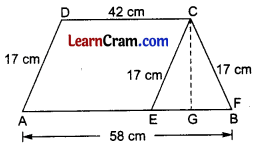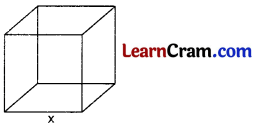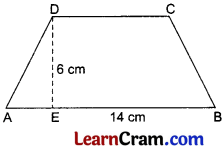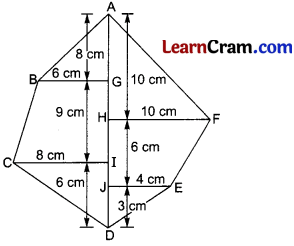# DAV Class 8 Maths Chapter 14 Worksheet 1 Solutions

The DAV Maths Book Class 8 Solutions Pdf and DAV Class 8 Maths Chapter 14 Worksheet 1 Solutions of Mensuration offer comprehensive answers to textbook questions.

## DAV Class 8 Maths Ch 14 WS 1 Solutions

Question 1.
Find the area of a trapezium whose parallel sides are 57 cm and 33 cm and the distance between them is 13 cm.
Solution:
Area of trapezium = $$\frac{1}{2}$$ × (sum of parallel sides) × height
= $$\frac{1}{2}$$ × (57 + 33) × 13
= $$\frac{1}{2}$$ × 90 × 13
= 45 × 13
= 585 cm2Question 2.
The area of a trapezium is 850 sq. cm. One of the parallel sides is 64 cm and the perpendicular distance between the parallel sides is 17 cm. Find the length of the other side.
Solution:
Area of the trapezium = $$\frac{1}{2}$$ × (sum of || sides) × height
⇒ 850 = $$\frac{1}{2}$$ × (64 + 6) × 17
⇒ $$\frac{850 \times 2}{17}$$ = 64 + b
⇒ 100 = 64 + b
⇒ b = 100 – 64 = 36 cm
Hence, the length of other side = 36 cm

Question 3.
The area of a trapezium is 1586 cm2 and the sum of parallel sides is 122 cm. Find the distance between two parallel sides.
Solution:
Area of trapezium = $$\frac{1}{2}$$ × (sum of || sides) × height
⇒ 1586 = $$\frac{1}{2}$$ × 122 × height
⇒ height = $$\frac{1586 \times 2}{122}$$ = 26 cm
Hence, the distance between the parallel sides = 26 cm

Question 4.
If the area of a trapezium is 28 m2 and two parallel sides are 8 m and 60 dm respectively, find the altitude.
Solution:
Area of trapezium = $$\frac{1}{2}$$ × (sum of || sides) × height
⇒ 28 = $$\frac{1}{2}$$ × (8 + 6) × height (∵ 1 m = 10 dm)
⇒ 28 = $$\frac{1}{2}$$ × 14 × height
⇒ height = 4 m
Hence, the height or altitude = 4 mQuestion 5.
Find the height of a trapezium whose area is 1080 cm2 and lengths of its parallel sides are 55.6 cm and 34.4 cm.
Solution:
Area of trapezium = $$\frac{1}{2}$$ × (sum of || sides) × height
⇒ 1080 = $$\frac{1}{2}$$ × (55.6 + 34.4) × height
⇒ 1080 = $$\frac{1}{2}$$ × 90 × height
⇒ height = $$\frac{1080}{45}$$ = 40 cm
Hence, the required height = 40 cm

Question 6.
The area of a trapezium is 12 sq. m. If its height is 3 m, find the sum of its parallel sides.
Solution:
Area of trapezium = $$\frac{1}{2}$$ × (sum of || sides) × height
⇒ 12 = $$\frac{1}{2}$$ × (sum of || sides) × 3
⇒ sum of || sides = $$\frac{12 \times 2}{3}$$
⇒ sum of || sides = 8 cm
Hence, the required sum of parallel sides = 8 cm.

Question 7.
The area of a trapezium is 248 sq. m and its height is 8 m. If one of the parallel sides is smaller than the other by 4 m, find two parallel sides.
Solution:
Let the smaller side be x m.
∴ Other side = (x + 4) m
∴ Area of the trapezium = $$\frac{1}{2}$$ × (sum of parallel sides) × height
⇒ 248 = $$\frac{1}{2}$$ × (x + x + 4) × 8
⇒ $$\frac{248 \times 2}{8}$$ = 2x + 4
⇒ 62 = 2x + 4
⇒ 2x = 58
⇒ x = 29
Hence, the smaller side = 29 m, and the other side = 29 + 4 = 33 m.Question 8.
Find the two parallel sides of a trapezium whose area is 1.6 m2, altitude is 10 dm and one of the parallel sides is longer than the other by 8 dm.
Solution:
Let one of the parallel sides be x m
∴ other side = (x + 0.8) m [∵ 1 m = 10 dm]
Area of the trapezium = $$\frac{1}{2}$$ × (sum of parallel sides) × altitude
⇒ 1.6 = $$\frac{1}{2}$$ × (x + x + 0.8) × 1
⇒ 1.6 × 2 = 2x + 0.8
⇒ 3.2 = 2x + 0.8
⇒ 3.2 – 0.8 = 2x
⇒ 2.4 = 2x
⇒ x = 1.2
Hence, the parallel sides are 1.2 m and 1.2 + 0.8 = 2 m.

Question 9.
The cross-section of a canal is in the form of a trapezium. If the top of the canal is 15 m wide, the bottom is 8 m and the area of cross-section is 138 m2, find its depth.
Solution:
Area of trapezium = $$\frac{1}{2}$$ × (sum of parallel sides) × height
⇒ 138 = $$\frac{1}{2}$$ × (15 + 8) × h
⇒ 138 = $$\frac{1}{2}$$ × 23 × h
⇒ h = $$\frac{138 \times 2}{23}$$ = 12 m
Hence, the depth of the canal is 12 m.Questions 10.
Two parallel sides of a trapezium are 58 cm and 42 cm. The other two sides are of equal length which is 17 cm. Find the area of the trapezium.
Solution:In the figure given alongside, draw $$\overline{\mathrm{DA}} \| \overline{\mathrm{CE}}$$.
∴ AECD is a parallelogram.
∴ $$\overline{\mathrm{CE}}$$ = $$\overline{\mathrm{CB}}$$ = 17 cm
$$\overline{\mathrm{EB}}$$ = 58 cm – 42 cm = 16 cm
∴ $$\overline{\mathrm{EG}}$$ = 8 cm
In right ΔCEG,
CE2 = EG2 + CG2
⇒ (17)2 = (8)2 + CG2
⇒ 289 = 64 + CG2
⇒ CG2 = 289 – 64 = 225
⇒ CG = $$\sqrt{225}$$ = 15 cm
∴ Area of trapezium = $$\frac{1}{2}$$ × (sum of parallel sides) × height
= $$\frac{1}{2}$$ × (58 + 42) × 15
= $$\frac{1}{2}$$ × 100 × 15
= 50 × 15
= 750 cm2
Hence, the required area = 750 cm2

Question 11.
The lengths of the parallel sides of a trapezium are in the ratio 4 : 7. If the height of the trapezium is 14 m and its area is 385 sq.m, find the length of the parallel sides.
Solution:
Let the length of the parallel sides be 7x cm and 4x cm.
∴ Area of trapezium = $$\frac{1}{2}$$ × (sum of parallel sides) × height
⇒ 385 = $$\frac{1}{2}$$ × (4x + 7x) × 14
⇒ 11x = $$\frac{385 \times 2}{14}$$
⇒ 11x = 55
⇒ x = 5
Hence, the length of parallel sides is 4 × 5 = 20 cm and 7 × 5 = 35 cm.

Question 12.
The perimeter of a trapezium is 104 m, its non-parallel sides are 18 m and 22 m, and its altitude is 16 m. Find the area of the trapezium.
Solution:
Sum of parallel sides = 104 – (18 + 22)
= 104 – 40
= 64 m
Area of the trapezium = $$\frac{1}{2}$$ × (sum of the parallel sides) × altitude
= $$\frac{1}{2}$$ × 64 × 16
= 512 cm2
Hence, the required area = 512 cm2### DAV Class 8 Maths Chapter 14 Worksheet 1 Notes

1. Trapezium:
A pair of opposite sides are parallel.$$\overline{\mathrm{AB}} \| \overline{\mathrm{DC}}$$, $$\overline{\mathrm{DE}} \perp \overline{\mathrm{AB}}$$ and $$\overline{\mathrm{CF}} \perp \overline{\mathrm{AB}}$$
Area of Trapezium ABCD = $$\frac{1}{2}(\overline{\mathrm{AB}}+\overline{\mathrm{CD}}) \times \overline{\mathrm{DE}}$$ABCD is a general quadrilateral in which $$\overline{\mathrm{AC}}$$ is diagonal, $$\overline{\mathrm{DE}} \perp \overline{\mathrm{AC}}$$ and $$\overline{\mathrm{DF}} \perp \overline{\mathrm{AC}}$$
∴ Area of □ABCD = $$\frac{1}{2} \times \overline{\mathrm{AC}} \times(\overline{\mathrm{DE}}+\overline{\mathrm{BF}})$$3. Cube:
Let x be the edge of the cube.∴ Volume = x3 cm3
Total surface area = 6x2 cm2
Length of the diagonal = √3x cm

4. Cuboid:Volume = l × b × h cm3
Total surface area = 2 [lb + bh + 1h] cm2
Length of the diagonal = $$\sqrt{l^2+b^2+h^2}$$ cm

5. Cylinder:Volume = πr2 h
Curved surface area = 2πrh
Total surface area = 2πrh + 2πr2 = 2πr (h + r)Example 1.
The parallel sides of a trapezium are 10 cm and 12 cm which its height is 6 cm. Find its area.
Solution:Area of trapezium = $$\frac{1}{2}$$ (sum of || sides × height)
= $$\frac{1}{2}(\overline{\mathrm{AB}}+\overline{\mathrm{DC}}) \times \overline{\mathrm{DE}}$$
= $$\frac{1}{2}$$ (12 + 10) × 6
= latex]\frac{1}{2}[/latex] × 22 × 6
= 66 cm2.

Example 2.
One of the parallel sides of a trapezium is 14 cm and its height is 6 cm. If its area is 66 cm2, find its other side.
Solution:Area of trapezium = $$\frac{1}{2}$$ (sum of || sides) × height
⇒ 66 = $$\frac{1}{2}$$ (14 + $$\overline{\mathrm{DC}}$$) × 6
⇒ $$\frac{2 \times 66}{6}$$ = (14 + $$\overline{\mathrm{DC}}$$)
⇒ 22 = 14 + $$\overline{\mathrm{DC}}$$
∴ $$\overline{\mathrm{DC}}$$ = 22 – 14 = 8 cm.Example 3.
Find the area of a trapezium whose parallel sides are 15 cm and 12 cm and other sides are 10 cm each,
Solution:Solution:
Draw $$\overline{\mathrm{CE}} \| \overline{\mathrm{DA}}$$ and $$\overline{\mathrm{CF}} \perp \overline{\mathrm{AB}}$$.
∴ □ ABCD is a parallelogram in which $$\overline{\mathrm{AD}}=\overline{\mathrm{CE}}$$ = 10 cm
and $$\overline{\mathrm{AE}}=\overline{\mathrm{DC}}$$ = 12 cm
$$\overline{\mathrm{EB}}$$ = 24 – 12 = 12 cm
$$\overline{\mathrm{EF}}$$ = $$\frac{12}{2}$$ = 6 cm
$$\overline{\mathrm{CF}}=\sqrt{\mathrm{CE}^2-\mathrm{EF}^2}$$
= $$\sqrt{(10)^2-(6)^2}$$
= $$\sqrt{100-36}=\sqrt{64}$$
= 8 cm
∴ Area of the trapezium = $$\frac{1}{2}$$ (AB + DC) × CF
= $$\frac{1}{2}$$ (24 + 12) × 8
= $$\frac{1}{2}$$ × 36 × 8
= 144 cm2.

Example 4.
Find the area of the given polygon with the given measurement.Solution:
Area of the polygon ABCDEF = ar (ΔGB) + ar(Trap BGIC) + ar(ΔCID) + ar(ΔDJE) + ar(Trap HJEF) + ar(ΔAHF)
= $$\frac{1}{2}$$ × 6 × 8 + $$\frac{1}{2}$$ (6 + 8) × 9 + $$\frac{1}{2}$$ × 8 × 6 + $$\frac{1}{2}$$ × 3 × 4 + $$\frac{1}{2}$$ (4 + 10) × 6 + $$\frac{1}{2}$$ × 10 × 10
= 24 + 63 + 24 + 6 + 42 + 50
= 211 cm2Example 5.
If the volume of a cube is 64 cm3, find its surface area and its diagonal,
Solution:
V = x3
64 = x3
∴ x = (64)1/3 = 4 cm
Surface area = 6x2
= 6 × (4)2
= 6 × 4 × 4
= 96 cm2

Length of diagonal = √3x
= √3 × 4
= 4√3 cm

Example 6.
The length, breadth and height of a cuboid are in the ratio 3 : 2 : 1. If its volume is 48 cm3, find its total surface area.
Solution:
Let the length, breadth and height of the cuboid be 3x cm, 2x cm and x cm.
∴ Volume = l × b × h
48 = 3x × 2x × x
48 = 6x3
x3 = 8
∴ x = $$\sqrt{8}$$ = 2
∴ l = 3 × 2 = 6 cm,
b = 2 × 2 = 4 cm
and h = 2 cm
Total surface area of the cuboid = 2[lb + bh + lh]
= 2 [6 × 4 + 4 × 2 + 2 ×x 6]
= 2 [24 + 8 + 12]
= 2 × 44 = 88cm2Example 7.
The volume of a right circular cylinder is 308 cm3. If its height is 18 cm, find its radius and total surface area.
Solution:
V = πr2h
308 = πr2 × 18
r2 = $$\frac{308 \times 7}{22 \times 18}$$
r2 = $$\frac{49}{4}$$ cm
∴ r = $$\frac{7}{3}$$ cm.
Total Surface Area (T.SA.) = 2πr (h + r)
= 2 × $$\frac{22}{7} \times \frac{7}{3}\left(18+\frac{7}{3}\right)$$
= 2 × $$\frac{22}{7} \times \frac{7}{3} \times \frac{61}{3}$$
= $$\frac{2684}{9}$$
= 298 $$\frac{2}{9}$$ cm2
Hence, r = $$\frac{7}{3}$$ cm and
T.S.A. = 298 $$\frac{2}{9}$$ cm2